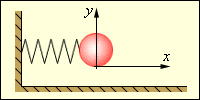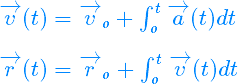# Frame dependency of accelerations

• I
• Masterov
In summary, the relativistic effects are visual only if you are looking at an object from a specific angle.f

#### Masterov

No. Length contraction can be seen as a result of viewing a 4d object at a different angle. It's closely analogous to the fact that you can slice a sausage perpendicular to its length and get a circular face, or at an angle and get an elliptical face. The sausage hasn't changed (and certainly spacetime hasn't), but the part of it you are looking at has.
Does this mean that the relativistic effects are visual?

Does this mean that the relativistic effects are visual?
No. Any experiment, optical or mechanical or whatever, will yield the same (contracted) length.

Any?

Experiment (for example):

We will measure acceleration a(t):The acceleration is the absolute,
and it can be measured by
the mass on the spring.

The actual position and speed:Acceleration is not absolute. Everyone agrees whether someone is undergoing proper acceleration or not. They do not agree on the magnitude of the 3-acceleration, which is what you are integrating.

Acceleration is not absolute?

Mass on the spring shows the same acceleration, regardless of the speed of the inertial system.

Or no?

No. ##F=\gamma^3 ma##, assuming velocity and acceleration are parallel. If what you said were true, a 1N force applied to a 1kg mass going 1m/s below c would exceed light speed in one second (thanks to @Dale for that example).

The acceleration experienced by an astronaut depends on the velocity of the observer.

So?

It's hard to imagine it.

google translate not cope with the transformation.
======================================

Mass on the spring shows the same acceleration, regardless of the speed of the inertial system.

Or no?
A mass on a spring doesn't measure acceleration; it measures force in the frame in which the mass is (momentarily) at rest. You can convert this to proper acceleration if you know the mass. It doesn't measure force in any other frame.

You are mixing up frames.

In the astronaut's rest frame then his acceleration is his proper acceleration and is an invariant.

However, in any other frame, the measured acceleration depends on the astronaut's velocity relative to that frame.

The second one is the relevant point of view if we are discussing length contraction. In the first case we would measure proper length and proper acceleration.

The acceleration experienced by an astronaut depends on the velocity of the observer.

So?

It's hard to imagine it.
Probably hard to image because you are misinterpreting what was said, and setting up a false straw man argument. No one said the astronaut him/herself did not EXPERIENCE a specific acceleration, what was said that other frames of reference do not agree that it is the same. The astronaut doesn't care what they see, just as neither I nor my clock care that we are seen as MASSIVELY time dilated by a particle in CERN.

EDIT: I see Ibix corrected you before I finished typing.

It does not. However, that is not relevant to the thread(Edit: not relevant to the topic of length contraction, which was the topic of the thread this was orignially posted in), nor to the point you were trying to make with your mass on a spring.

Last edited:
Weight of astronaut (in outer space), whith he presses on a seat, do not depend on the velocity of the observer.

With this you agree?

The acceleration experienced by an astronaut depends on the velocity of the observer. Or no?
If by "acceleration" you mean "proper acceleration" (which is what the astronaut feels pushing on him, but has very little to do with his or anyone else's measurement of how his speed is changing) then the answer is "no"

If by "acceleration" you mean coordinate acceleration (how his speed is changing with time) then the answer is "yes".

Does this mean that the relativistic effects are visual?
Any?

Experiment (for example):

We will measure acceleration a(t):The acceleration is the absolute,
and it can be measured by
the mass on the spring.

The actual position and speed:======================================

The acceleration experienced by an astronaut depends on the velocity of the observer.

Or no?

Good by...

Obviously ##\vec{a}(t)## is only a function of time.

Actually, on second thought, this isn't so obvious! But we write a(t), and not a(t,v), so in this sense, a(t) is only a function of time.

While it's clear that ##\vec{a}(t)## is in some only a function of time, It's not clear what you mean by "the acceleration experienced by an astronaut". One presumes that we are invited to imagine that you are asking about ##\vec{a}(t)##, but the presence of the unexplained spring and mass in your diagram and the wording of your question suggests you might be interested in something other than ##\vec{a}##.

Several people have talked about things you might have mean by "the acceleration experienced by the astronaut" at length, but you've refused to clarify exactly what you meant, and instead of honoring the repeated requests to clarify your quesiton, you refuse to clarify and demanded a yes or no response to an unclear question. This is rather unfortunate.

Additionally, if we have a frame S, and a frame S', and frame S' is moving relative to frame S with a velocity v, we can talk about a(t) in frame S, and a'(t') in frame S', and ask the question - does the transform between a(t) and a'(t') depend on the relative velocity v between S and S'? The answer in special relativity is yes, unlike the answer in Newtonian mechanics.

Last edited:
Well, let's calculate! To discuss Lorentz-transformation properties of physical observables it's good to use manifestly covariant descriptions. This leads quite naturally to the use of the proper time as time variable. Then momentum is
$$p^{\mu}=m \frac{\mathrm{d} x^{\mu}}{\mathrm{d} \tau}.$$
Since by definition (##c=1##, natural units)
$$\mathrm{d} \tau^2 = \mathrm{d} x^{\mu} \mathrm{d} x_{\nu}$$
you have the mass-shell condition
$$p_{\mu} p^{\mu}=m^2=\text{const},$$
and ##m## is the invariant mass (a Lorentz scalar).

The covariant equations of motion reads
$$\frac{\mathrm{d} p^{\mu}}{\mathrm{d} \tau}=\dot{p}^{\mu} = K^{\mu},$$
where ##K^{\mu}## is the Lorentz-force four-vector. Because of the mass-shell condition you have
$$p_{\mu} \dot{p}^{\mu}=0 \; \Rightarrow \; K^{\mu} p_{\mu}=0.$$
This constraint makes only three of the four components of the equations of motion independent, as it should be, i.e., you can solve the three spatial equations, and then the temporal one (which is nothing but the relativistic work-energy theorem) is fulfilled automatically.

A typical example for a Lorentz four-force is the force on a charged particle in an electromagnetic field
$$K_{\mu}=\frac{q}{m} F_{\mu \nu} p^{\nu},$$
where ##F_{\mu \nu}## is the antisymmetric field-strength tensor (or Faraday tensor) with the components of the electric and magnetic field. Then of course the constraint is fulfilled.

The covariant acceleration
$$a^{\mu}=\frac{1}{m} \dot{p}^{\mu}=\ddot{x}^{\mu}$$
is obviously a four-vector and transforms as such under Lorentz transformations. Since ##a_{\mu} p^{\mu}=0## and ##p^{\mu}## is always a time-like vector ##a^{\mu}## is spacelike. Nevertheless, contrary to the situation in non-relativistic mechanics, the acceleration also changes its components under rotation-free Lorentz boosts.

•Ibix